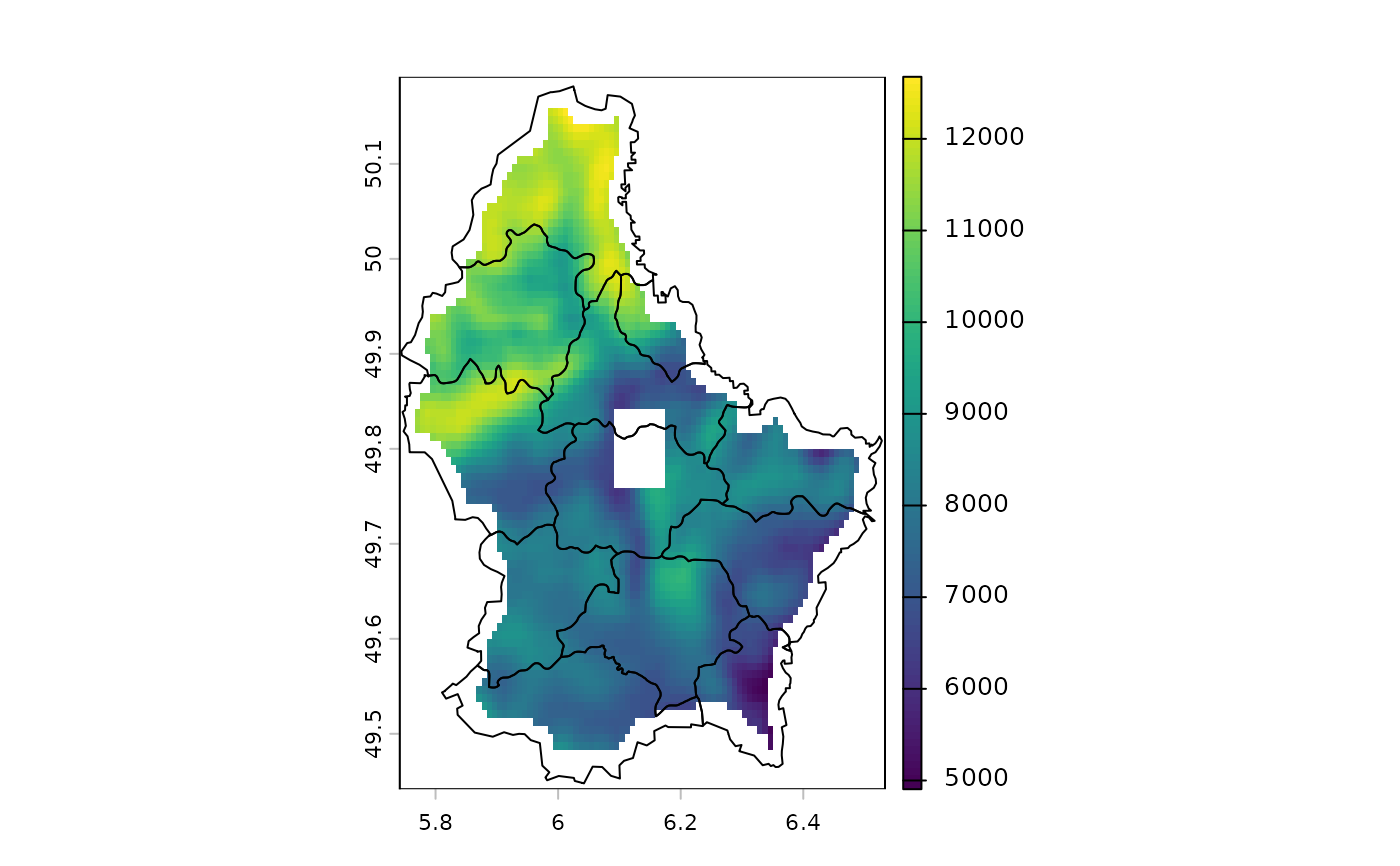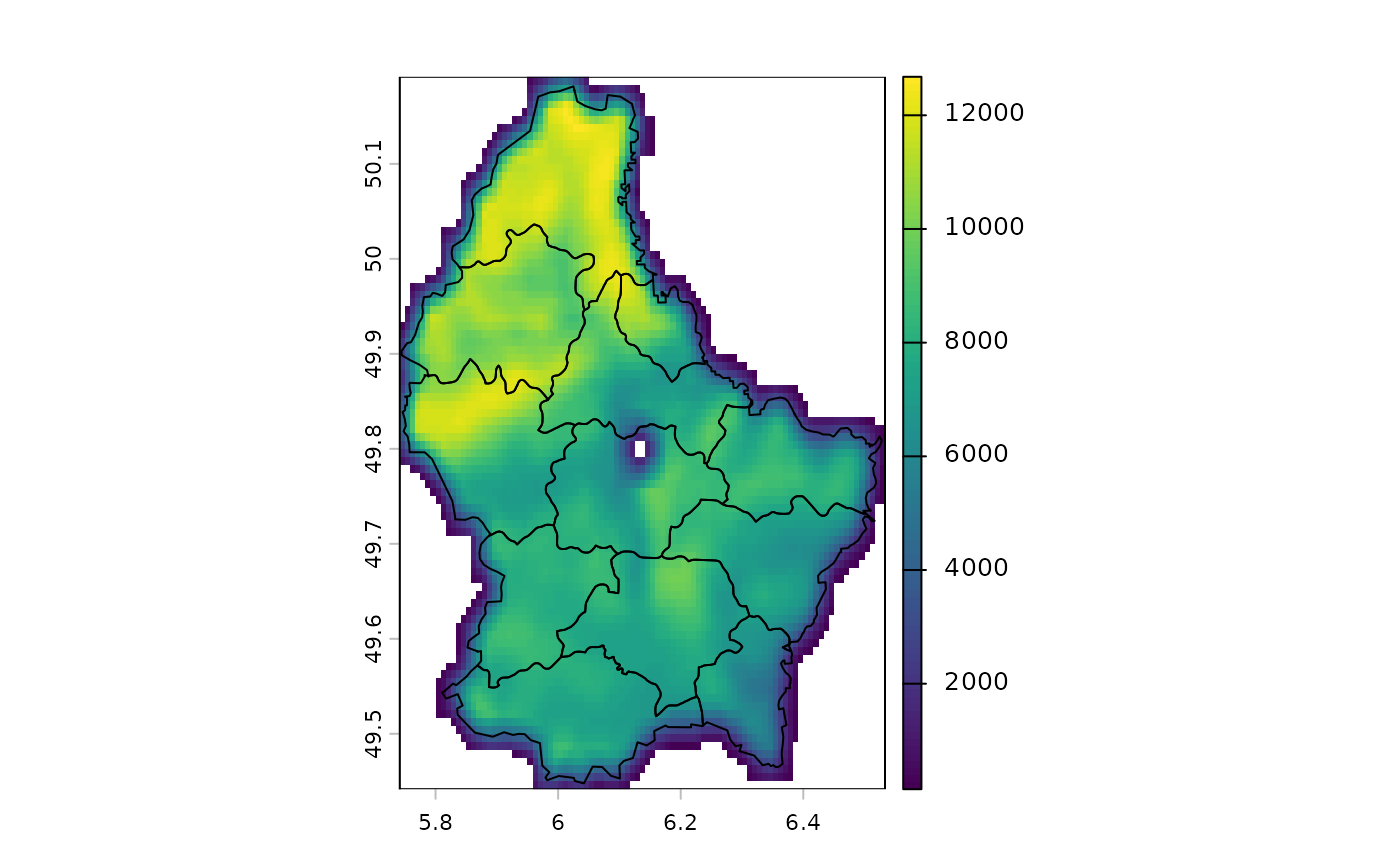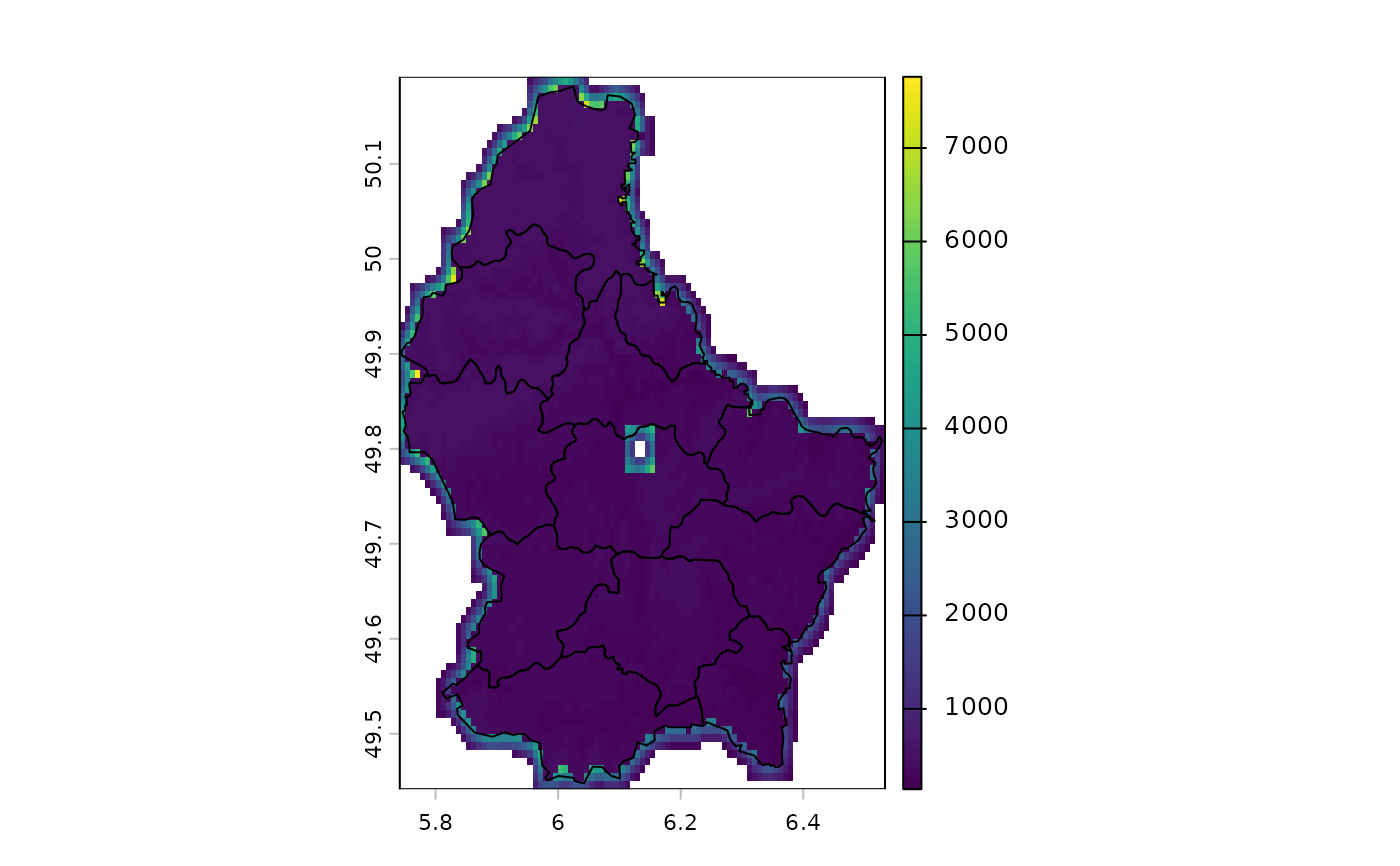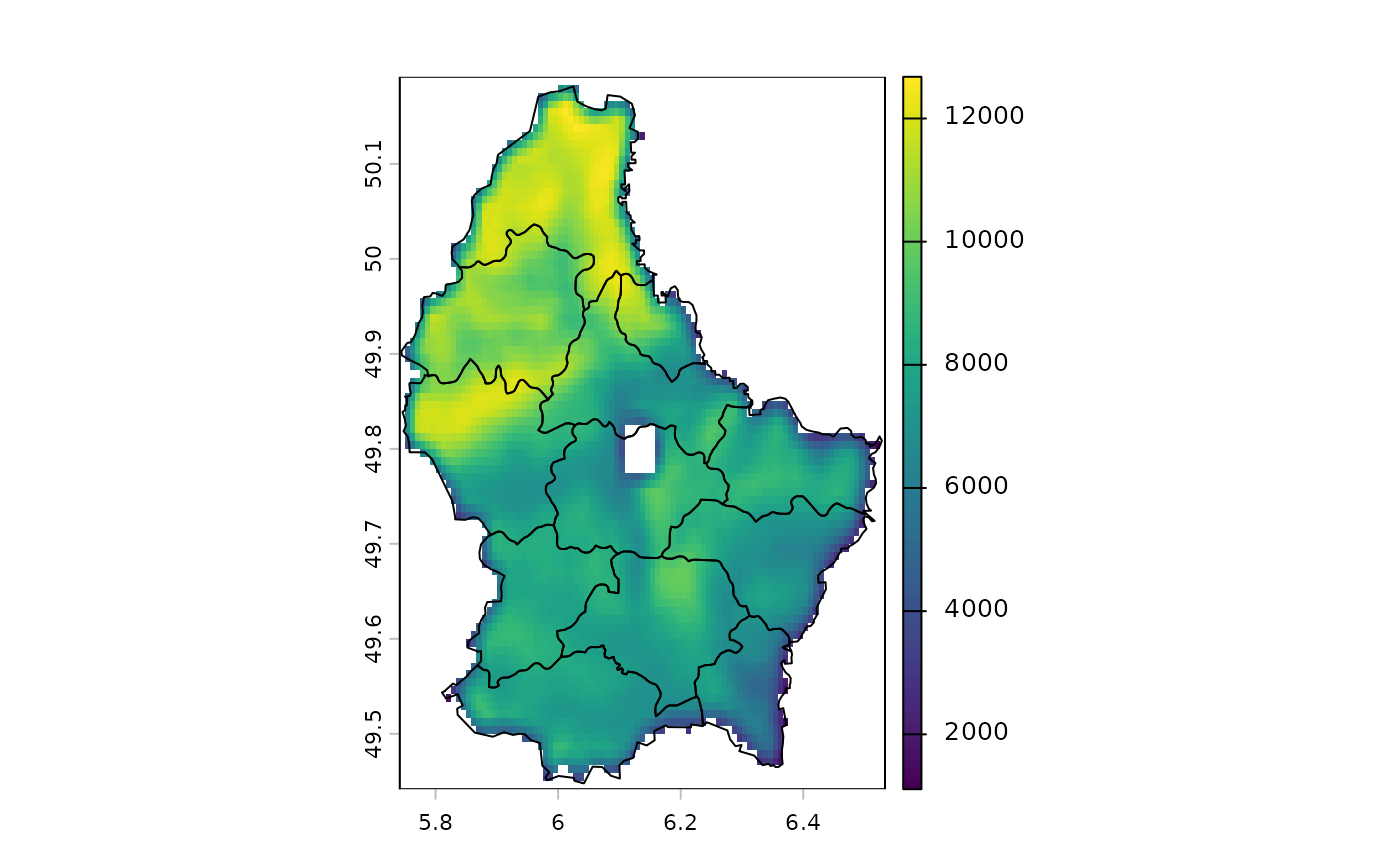Calculate focal ("moving window") values for each cell.

# S4 method for SpatRaster
focal(x, w=3, fun="sum", ..., na.policy="all", fillvalue=NA,
expand=FALSE, silent=TRUE, filename="", overwrite=FALSE, wopt=list())

## Arguments

x

SpatRaster

w

window. The window can be defined as one (for a square) or two numbers (row, col); or with an odd-sized weights matrix. See Details.

fun

function that takes multiple numbers, and returns a numeric vector (one or multiple numbers). For example mean, modal, min or max

...

additional arguments passed to fun such as na.rm

na.policy

character. Can be used to determine the cells of x for which focal values should be computed. Must be one of "all" (compute for all cells), "only" (only for cells that are NA) or "omit" (skip cells that are NA). Note that the value of this argument does not affect which cells around each focal cell are included in the computations (use na.rm=TRUE to ignore cells that are NA for that)

fillvalue

numeric. The value of the cells in the virtual rows and columns outside of the raster

expand

logical. If TRUE The value of the cells in the virtual rows and columns outside of the raster are set to be the same as the value on the border. Only available for "build-in" funs such as mean, sum, min and max

silent

logical. If TRUE error messages are printed that may occur when trying fun to determine the length of the returned value. This can be useful in debugging a fun that does not work

filename

character. Output filename

overwrite

logical. If TRUE, filename is overwritten

wopt

additional arguments for writing files as in writeRaster

## Details

focal The window used must have odd dimensions. If you need even sides, you can use a matrix and add a column or row with weights of zero.

Window values are typically 0 or 1, or a value between 0 and 1 if you are using a rectangular area and/or the "sum" function. They can also be NA; these are ignored in the computation. That can be useful to compute, for example, the minimum or maximum value for a non-rectangular area.

The "mean" function is a special case, as zero weights are ignored automatically.

The "sum" function returns NA if all focal cells are NA and na.rm=TRUE. R would normally return a zero in these cases. See the difference between focal(x, fun=sum, na.rm=TRUE) and focal(x, fun=\(i) sum(i, na.rm=TRUE))

Example weight matrices

Laplacian filter: filter=matrix(c(0,1,0,1,-4,1,0,1,0), nrow=3)

Sobel filters (for edge detection):

fx=matrix(c(-1,-2,-1,0,0,0,1,2,1), nrow=3)

fy=matrix(c(1,0,-1,2,0,-2,1,0,-1), nrow=3)

## Value

SpatRaster

focalMat, focalValues, focal3D, focalPairs, focalReg, focalCpp

## Examples

r <- rast(ncols=10, nrows=10, ext(0, 10, 0, 10))
values(r) <- 1:ncell(r)

f <- focal(r, w=3, fun=function(x, ...) quantile(x, c(.25, .5, .75), ...), na.rm=TRUE)

f <- focal(r, w=3, fun="mean")

# the following two statements are equivalent:
a <- focal(r, w=matrix(1/9, nc=3, nr=3))
b <- focal(r, w=3, fun=mean, na.rm=FALSE)

# but this is different
d <- focal(r, w=3, fun=mean, na.rm=TRUE)

## illustrating the effect of different
## combinations of na.rm and na.policy
v <- vect(system.file("ex/lux.shp", package="terra"))
r <- rast(system.file("ex/elev.tif", package="terra"))
r[45:50, 45:50] <- NA

# also try "mean" or "min"
f <- "sum"
# na.rm=FALSE
plot(focal(r, 5, f) , fun=lines(v))# na.rm=TRUE
plot(focal(r, 5, f, na.rm=TRUE), fun=lines(v))# only change cells that are NA
plot(focal(r, 5, f, na.policy="only", na.rm=TRUE), fun=lines(v))# do not change cells that are NA
plot(focal(r, 5, f, na.policy="omit", na.rm=TRUE), fun=lines(v))# does not do anything
# focal(r, 5, f, na.policy="only", na.rm=FALSE)